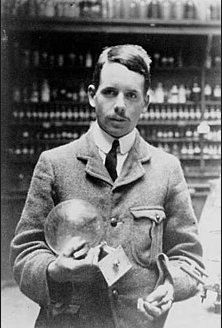Mendeleev arranged the elements according to increasing atomic masses, but he was forced to change the position of some of them to form groups with similar properties. In 1913, Moseley observed that the frequencies of X-rays emitted by an element were related to the number of positive charges present in the nucleus (protons), according to the equation $\nu=A(Zb)^2$, where $\ nu$ the frequency of X-rays, Z the atomic number, A and B constants.
In this way, he managed to predict the existence of three new elements, with atomic numbers Z=43, 61 and 45. From Moseley's work, the elements were ordered by atomic number and not by atomic mass.
Elements with similar properties, when ordered by atomic number, are located in the same column (group).